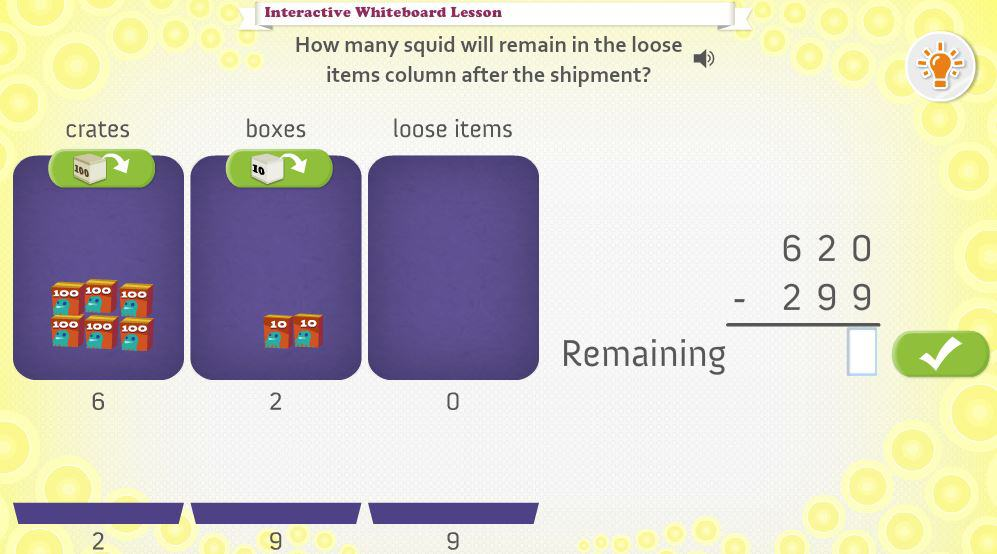# Interactive Subtraction Algorithm (4th Grade)

Dream Box - Subtraction Algorithm.  Students use the standard subtraction algorithm and a place value workspace to solve subtraction problems involving up to two 3-digit numbers.  LessonCCSS.MATH.CONTENT.4.NBT.B.4
Fluently add and subtract multi-digit whole numbers using the standard algorithm.